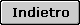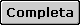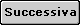<% If strDisplayBorderYN = SUR_BOOLEAN_NEGATIVE Then Response.Write "      " End If %><%=strTitle%><%=strTitle%> <%=strTitle%><%=strTitle%>
<% 'Optionally display the border If strDisplayBorderYN = SUR_BOOLEAN_POSITIVE Then %> ">
<% Else %> ">
<% End If %> <% 'Only display the page number if a page numbering format was selected If rsSurvey("page_numbering_format") <> SUR_PAGE_NUMBERING_DO_NOT_DISPLAY Then %> <% Else %> <% End If 'Only display the page title if there is one If Len(strPageTitle) > 0 Then %> <% End If 'Only display the page introduction if there is one If Len(strPageIntroduction) > 0 Then %> <% End If %>
<% 'Display the page numbers in the appropriate format If rsSurvey("page_numbering_format") = SUR_PAGE_NUMBERING_DISPLAY_PROGRESS Then %> Pagina <%=lngActualPageNumber%> di <%=lngPageCount%> <% ElseIf rsSurvey("page_numbering_format") = SUR_PAGE_NUMBERING_DISPLAY_PROGRESS_BAR Then %>
 Pagina <%=lngActualPageNumber%> di <%=lngPageCount%>
<% Else 'SUR_PAGE_NUMBERING_DISPLAY_PAGE_NUMBER_ONLY %> Pagina <%=lngActualPageNumber%> <% End If %>

<%=strPageTitle%>
<%=strPageIntroduction%>

<% If Request.Form("AdminOrOwnerEditResponse") = SUR_BOOLEAN_POSITIVE_DISPLAY Or Request.QueryString("Edit") = SUR_BOOLEAN_POSITIVE_DISPLAY Then strEditValue = SUR_BOOLEAN_POSITIVE_DISPLAY Else strEditValue = SUR_BOOLEAN_NEGATIVE_DISPLAY End If %> " method="post" name="frmSurvey"> " name="EID"> <% If InStr(Request.ServerVariables("QUERY_STRING"), "ItemID") > 0 Then For Each strTempValue In Request.Querystring If Left(strTempValue, 6) = "ItemID" Then strItemIDValues = strItemIDValues & strTempValue & "=" & Request.Querystring(strTempValue) & "&" End If Next Else strItemIDValues = "" End If %> " name="PostedQueryString"> <% If SUR_DATABASE_TYPE = "SQLServer" Or SUR_DATABASE_TYPE = "MySQL" Then strTempDate = Year(Now()) & SUR_DATE_DELIMITER & Month(Now()) & SUR_DATE_DELIMITER & Day(Now()) Else If SUR_DATE_FORMAT = SUR_DATE_FORMAT_AMERICAN Then strTempDate = Month(Now()) & SUR_DATE_DELIMITER & Day(Now()) & SUR_DATE_DELIMITER & Year(Now()) Else 'SUR_DATE_FORMAT_EUROPEAN strTempDate = Day(Now()) & SUR_DATE_DELIMITER & Month(Now()) & SUR_DATE_DELIMITER & Year(Now()) End If End If %> " name="ResponseStartDate"> " name="hdnUpdatingSurvey"> <% 'Add a value to SESSION indicating the survey and page number the user is about to take. On the "action" 'page for this survey, check this value again to make sure that the same page is not submitted twice. Session(SUR_UNIQUE_RESPONSE_IDENTIFIER) = CStr(lngSurveyID) & CStr(lngActualPageNumber) strQuestionNumberingFormat = rsSurvey("question_numbering_format") Do While Not rsSurvey.EOF %> <% rsSurvey.MoveNext Loop 'If the question numbering format is within each page, update the next question number to 1, since that is 'the number each page will always start with. Otherwise, leave the variable lngQuestionNumber as is, which 'will cause the next page to be started with the subsequent number in the sequence. If strQuestionNumberingFormat = SUR_QUESTION_NUMBERING_EACH_PAGE Then lngQuestionNumber = 1 End If %>
 <% 'Display the back button on all but the first page, but only if the back button should be displayed, 'based on the settings for the survey. If lngActualPageNumber <> 1 And strBackButtonDisplay = SUR_BACK_BUTTON_DISPLAY Then 'Use the button for the Back button, if provided If Len(strNavigationBackButtonPath) > 0 Then 'Use the link text for the Back button, if provided If Len(strNavigationBackButtonPath) > 0 Then %><% Else %><% End If Else %> <% End If End If 'If this is the last page, display a "Done" button; otherwise, display a "Next" button. If CLng(lngPageCount) = CLng(lngActualPageNumber) Then 'Use the button for the Next button, if provided If Len(strNavigationDoneButtonPath) > 0 Then 'Use the button path provided, if there is one; otherwise, use the default button If Len(strNavigationDoneButtonPath) > 0 Then %><% Else %><% End If Else %> <% End If Else 'Use the button for the Next button, if provided If Len(strNavigationNextButtonPath) > 0 Then 'Use the button path provided, if there is one; otherwise, use the default button If Len(strNavigationNextButtonPath) > 0 Then %><% Else %><% End If Else %> <% End If End If 'Only display the Cancel button if it was not explicitly set to "Do not display" If strCancelButtonDisplay <> SUR_CANCEL_BUTTON_DO_NOT_DISPLAY Then 'If the button was provided for the Cancel button, use that first If Len(strNavigationCancelButtonPath) > 0 Then 'Use the button path provided, if there is one; otherwise, use the default button If Len(strNavigationCancelButtonPath) > 0 Then strButtonPath = strNavigationCancelButtonPath Else strButtonPath = "Resources/SurveyButtons/Cancel.gif" End If 'Set the appropriate action, based on the survey options, for what to do if a user clicks the Cancel button If strCancelButtonDisplay = SUR_CANCEL_BUTTON_CLOSE Then %><% Else 'Redirecting, so set a variable equal to the URL to redirect to If strCancelButtonDisplay = SUR_CANCEL_BUTTON_END_OF_SURVEY Then strRedirectURL = "TakeSurveyActionComplete.asp?SurveyID=" & EncryptSurveyID(lngSurveyID) Else 'SUR_CANCEL_BUTTON_REDIRECT strRedirectURL = strCancelButtonRedirectURL End If %><% End If Else 'Set the appropriate action for the Cancel button If strCancelButtonDisplay = SUR_CANCEL_BUTTON_CLOSE Then strRedirectURL = "javascript:window.close();" ElseIf strCancelButtonDisplay = SUR_CANCEL_BUTTON_END_OF_SURVEY Then strRedirectURL = "TakeSurveyActionComplete.asp?SurveyID=" & EncryptSurveyID(lngSurveyID) Else 'SUR_CANCEL_BUTTON_REDIRECT strRedirectURL = strCancelButtonRedirectURL End If %> <% End If End If %>